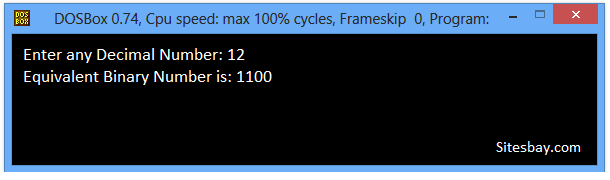# Convert Decimal to Binary Program in C++

## C++ Program to Convert Decimal Number to Binary Number

Decimal number is a base 10 number because it ranges from 0 to 9, there are total 10 digits between 0 to 9. Any combination of digits is decimal number such as 213, 385, 592, 2, 0, 8 etc.

Binary number is a base 2 number because it is either 0 or 1. Any combination of 0 and 1 is binary number such as 10101, 1001, 1111, 10010 etc.

With the help of C++ Language you can convert any number from decimal to binary and binary to decimal, binary to octal, binary to hexadecimal, decimal to octal and etc.

Before write this code you must be basic kanowledge about For Loop in C++, While Loop in C++ and Modulus Operator in C++.

### Decimal to Binary Conversion Algorithm

• Step 1: Divide the number by 2 through % (modulus operator) and store the remainder in array
• Step 2: Divide the number by 2 through / (division operator)
• Step 3: Repeat the step 2 until the number is greater than zero

## C++ Program to Convert Decimal to Binary

```
#include<iostream.h>
#include<conio.h>

void main()
{
int no,rem,i=0,j;
clrscr();
cout<<"Enter any Decimal Number: ";
cin>>no;
while(no>0)
{
rem[i]=no%2;
i++;
no=no/2;
}
cout<<"Equivalent Binary Number is: ";
for(j=i-1;j>=0;j--)
{
cout<<rem[j];
}
getch();
}
```

## Output

```Enter any Decimal Number: 12
Equivalent Binary Number is: 1100
```## Pure VPN Privide Lowest Price VPN Just @ \$1.65. Per Month with Non Detected IP Lowest Price Non Detected IP VPN